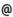## "Babes-Bolyai" University of Cluj-Napoca Faculty of Mathematics and Computer Science

 Ordinary differential equations and dynamical systems (1)
 Code Semes-ter Hours: C+S+L Credits Type Section ME001 3 2+2+0 6 compulsory Matematică ME001 3 2+2+0 6 compulsory Informatică ME001 5 2+2+0 5 compulsory Matematică-Informatică ME001 5 2+2+0 6 compulsory Matematici aplicate
 Teaching Staff in Charge
 Prof. PRECUP Radu, Ph.D., r.precupmath.ubbcluj.ro
 Prof. PETRUSEL Adrian Olimpiu, Ph.D., petruselmath.ubbcluj.ro
 Assoc.Prof. BEGE Antal, Ph.D., begemath.ubbcluj.ro
 Aims Introduction to the basic problems of ordinary differential equations control paper (25 % of the final mark), written examination (40%) and oral examination (35%).
 Content 1. Mathematical modelling and differential equations 2. Operatorial equations in metric spaces. 3. The Cauchy problem. 4. Solution set of a differential equation. 5. n-order linear differential equations 6. First order linear systems of differential equations
 References 1. A. RUS, Ecuatii diferentiale, ecuatii integrale si sisteme dinamice (Transilvania Press, Cluj-Napoca, 1996). 2. A. RUS, P. PAVEL, Ecuatii diferentiale, Ed. Didactica, Bucuresti, 1982. 3. V. BARBU, Ecuatii diferentiale, Ed. Junimea, Iasi, 1985. 4. D.V. IONESCU, Ecuatii diferentiale si integrale, Ed. Didactica, Bucuresti, 1972.
 Assessment Exam.